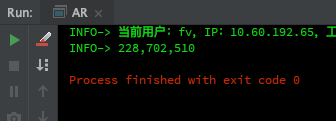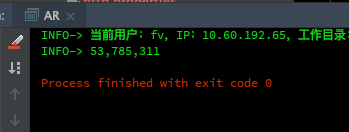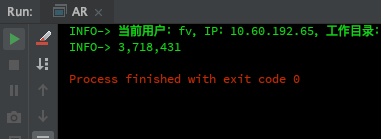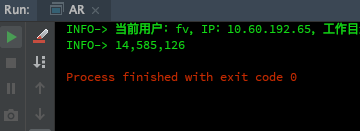# 如何测试这个方法--性能篇

 /**
* 生产唯一的交易订单号
*
* @return
*/
public static String createUniqueOrderNo() {
SimpleDateFormat nyrsfm = new SimpleDateFormat("yyyyMMddHHmmss");
return nyrsfm.format(new Date()) + getRandomLengthCode(4);
}

/**
* 获取随机的短信验证码
*
* @return
*/
public static String getRandomLengthCode(int length) {
return String.valueOf((int) ((Math.random() * 9 + 1) * Math.pow(10, length - 1)));
}


  static SimpleDateFormat nyrsfm = new SimpleDateFormat("yyyyMMddHHmmss");

/**
* 生产唯一的交易订单号
*
* @return
*/
public static String createUniqueOrderNo() {
return nyrsfm.format(new Date()) + getRandomLengthCode(4);
}


public static void main(String[] args) {
List<String> list = new ArrayList<>();
long nanoMark = getNanoMark();
range(10000).forEach(x ->
{
createUniqueOrderNo();
});
output(getFormatNumber(getNanoMark() - nanoMark));
}public static void main(String[] args) {
List<String> list = new ArrayList<>();
long nanoMark = getNanoMark();
range(100000).forEach(x ->
{
//            String ss = String.valueOf(1211);
String ss = 1121 + "";
});
output(getFormatNumber(getNanoMark() - nanoMark));
}posted @ 2019-12-08 14:08  FunTester  阅读(187)  评论(0编辑  收藏